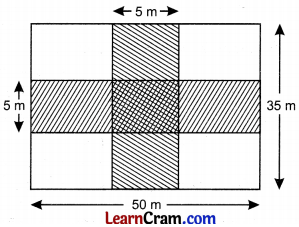# DAV Class 7 Maths Chapter 11 Worksheet 2 Solutions

The DAV Maths Class 7 Solutions and DAV Class 7 Maths Chapter 11 Worksheet 2 Solutions of Perimeter and Area offer comprehensive answers to textbook questions.

## DAV Class 7 Maths Ch 11 WS 2 Solutions

Question 1.
The given figure, shows two paths drawn inside a rectangular field 50 m long and 35 m wide. The width of the path is 5 m. Find the area of the shaded portion.Area of the path along the length of the rectangle = 50 × 5 = 250 m2
Area of the path along the width of the rectangle
= 35 × 5 = 175 m2
Area of the common portion = 5 × 5 = 25 m2
∴ Total area of the two paths (shaded)
= (250 m2 + 175 m2) – 25 m2
= 425 m2 – 25 m2
= 400 m2
Hence the required area = 400 m2.Question 2.
An oblong garden measures 60 m by 55 m. From the centre of each side, a path 2 meters wide goes across to the centre of the opposite side. Find the area of the paths.
Area of the path along length = 60 × 2 = 120 m2
Area of the path along breadth = 55 × 2 = 110 m2
Area of the common portion = 2 × 2 = 4 m2
Area of the paths = 120 m2 + 110 m2 – 4 m2
= 230 m2 – 4 m2
= 226 m2
Hence the area of the two path = 226 m2.

Question 3.
A rectangular plot of land is 300 m long and 250 m broad. It has two roads, each 3 m wide running midway within it, one parallel to the length and the other parallel to the breadth. Find the area of the roads. Also calculate the cost of constructing the roads at ₹ 50 per square meter.
Area of the road along the length of the plot
= 300 m × 3 m
= 900 m2

Area of the road along the width of the plot = 250 m × 3 m
= 750 m2
Area of the common portion = 3m × 3m
= 9m2

Area of the roads = 900 m2 + 750 m2 – 9 m2
= 1650 m2 – 9 m2
= 1641 m2

Cost of construction = 1641 × 50
= ₹ 82050
Hence the required area = 1641 m2 and the required cost = ₹ 82050.Question 4.
A rectangular piece of ground is 45 m long and 30 m wide. It has two roads each 1 m wide, running midway within it, one parallel to the length and the other parallel to the breadth. Find the area of roads. Also calculate the area of the remaining ground.